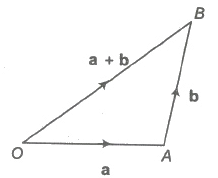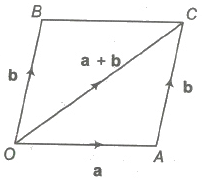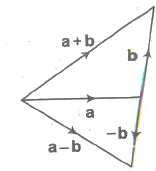JEE  >  Addition and Multiplication of a Vector by a Scalar

# Addition and Multiplication of a Vector by a Scalar - Mathematics (Maths) Class 12 - JEE

Let a and b be any two vectors. From the terminal point of a, vector b is drawn. Then, the vector from the initial point O of a to the terminal point B of b is called the sum of vectors a and b and is denoted by a + b. This is called the triangle law of addition of vectors.Parallelogram Law

Let a and b be any two vectors. From the initial point of a, vector b is drawn and parallelogram OACB is completed with OA and OB as adjacent sides. The vector OC is defined as the sum of a and b. This is called the parallelogram law of addition of vectors.

The sum of two vectors is also called their resultant and the process of addition as composition.(i) a + b = b + a (commutativity)

(ii) a + (b + c)= (a + b)+ c (associativity)

(iii) a+ O = a (additive identity)

(iv) a + (— a) = 0 (additive inverse)

(v) (k1 + k2) a = k1 a + k2a (multiplication by scalars)

(vi) k(a + b) = k a + k b (multiplication by scalars)

(vii) |a+ b| ≤ |a| + |b| and |a – b| ≥ |a| – |b|

Difference (Subtraction) of Vectors

If a and b be any two vectors, then their difference a – b is defined as a + (- b).Multiplication of a Vector by a Scalar

Let a be a given vector and λ be a scalar. Then, the product of the vector a by the scalar λ is λ a and is called the multiplication of vector by the scalar.

Important Properties

(i) |λ a| = |λ| |a|

(ii) λ O = O

(iii) m (-a) = – ma = – (m a)

(iv) (-m) (-a) = m a

(v) m (n a) = mn a = n(m a)

(vi) (m + n)a = m a+ n a

(vii) m (a+b) = m a + m b

The document Addition and Multiplication of a Vector by a Scalar | Mathematics (Maths) Class 12 - JEE is a part of the JEE Course Mathematics (Maths) Class 12.
All you need of JEE at this link: JEE

## Mathematics (Maths) Class 12

206 videos|264 docs|139 tests

## FAQs on Addition and Multiplication of a Vector by a Scalar - Mathematics (Maths) Class 12 - JEE

 1. What is a scalar?A scalar is a mathematical quantity that has magnitude but no direction. It is represented by a single number and can be positive, negative, or zero. Scalars are used in various mathematical operations, including addition and multiplication with vectors.
 2. How does scalar multiplication affect the direction of a vector?Scalar multiplication does not affect the direction of a vector. It only changes the magnitude of the vector. If the scalar is positive, the vector's magnitude will increase, and if the scalar is negative, the vector's magnitude will decrease. The direction of the vector remains unchanged.
 3. Can a vector be added to a scalar?No, a vector cannot be added to a scalar directly. Addition and multiplication of vectors and scalars are separate operations. Scalar addition is performed between two scalars, and vector addition is performed between two vectors of the same dimension.
 4. How is vector multiplication by a scalar different from scalar multiplication by a vector?Vector multiplication by a scalar involves multiplying each component of the vector by the scalar. The result is a new vector with the same direction but a different magnitude. On the other hand, scalar multiplication by a vector involves multiplying the scalar by each component of the vector. The result is a new vector with the same magnitude but a potentially different direction.
 5. Can scalar multiplication be applied to matrices?Yes, scalar multiplication can be applied to matrices. In this case, each element of the matrix is multiplied by the scalar, resulting in a new matrix with the same dimensions. The operation follows the same principles as scalar multiplication with vectors, where the scalar only affects the magnitude of the matrix elements, not their direction.

## Mathematics (Maths) Class 12

206 videos|264 docs|139 testsExplore Courses for JEE examSignup to see your scores go up within 7 days! Learn & Practice with 1000+ FREE Notes, Videos & Tests.
10M+ students study on EduRev
Track your progress, build streaks, highlight & save important lessons and more!
Related Searches

,

,

,

,

,

,

,

,

,

,

,

,

,

,

,

,

,

,

,

,

,

;